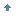# Prayer

posts - 1256, comments - 190, trackbacks - 0, articles - 0
C++博客 :: 首页 :: 新随笔 :: 联系 :: 聚合:: 管理
Awk 是一种优秀的文本样式扫描和处理工具。本文侧重介绍了 awk 在数值计算方面的运用，并通过几个实际工作中的例子，阐述了如何利用 awk 的计算功能来提高我们的工作效率。

Awk 是一种优秀的文本样式扫描和处理工具。 Awk 与 sed 和 grep 有些相似， 但功能比后者强不少。 awk 提供的功能包括样式载入， 流控制，数学运算符，进程控制以及许多内置的变量和函数等。 借助于这些功能， 我们可以很方便地利用 awk 对各种文件 （如试验产生的数据文件，数据库文件等） 进行处理。 本文介绍了 awk 在数值计算方面的运用， 并通过几个实际的例子， 阐述了如何利用 awk 的计算功能来提高我们的工作效率。

Awk 支持不少常见的运算符， 如 + （加），- （减）， * （乘）， / （除）， ^ 或 ** （乘方）， % （取模） 等等。 此外， awk 也提供了一些常用的数学函数, 比如 sin(x), cos(x), exp(x), log(x), sqrt(x), rand()。 使用这些运算符和函数可以直接进行一些简单的运算：

 ``` echo | awk '{print 19+7}' ==> 26 echo | awk '{print 19-7}' ==> 12 echo | awk '{print 19*7}' ==> 133 echo | awk '{print 19/7}' ==> 2.71429 echo | awk '{print 19**7}' ==> 893871739 echo | awk '{print 19%7}' ==> 5 echo | awk '{print atan2(19, 7)}' ==> 1.21781 ```

 ``` { print \$1 " + " \$2 " = " \$1 + \$2 print \$1 " - " \$2 " = " \$1 - \$2 print \$1 " x " \$2 " = " \$1 * \$2 print \$1 " / " \$2 " = " \$1 / \$2 print \$1 " ^ " \$2 " = " \$1 ** \$2 print \$1 " mod " \$2 " = " \$1 % \$2 print " atan2( " \$1 " , " \$2 " ) " " = " atan2(\$1, \$2) } ```回页首

 ```function fibo(n) { if(n<=1) return 1; return (fibo(n-2) + fibo(n-1)); } BEGIN { n = (ARGV < 1) ? 1 : ARGV; printf("%d\n", fibo(n)); exit; } ```

 ```function factorial(n) { if(n<=1) return 1; return (n*factorial(n-1)); } BEGIN { n = (ARGV < 1) ? 1 : ARGV; printf("%d\n", factorial(n)); exit; } ``````BEGIN { a = 3.7; x = a; while((x**2-a)**2 > 1e-12) { x = (x + a/x)/2; } print x } ```回页首

 ```File: "simu_space_1.dat" Size: 237928 Blocks: 480 IO Block: 4096 regular Datei Device: 801h/2049d Inode: 2768915 Links: 1 Access: (0644/-rw-r--r--) Uid: ( 1000/ nst) Gid: ( 1000/ nst) Access: 2008-11-14 10:56:05.000000000 +0100 Modify: 2008-11-13 23:26:44.000000000 +0100 Change: 2008-11-13 23:26:44.000000000 +0100 ```

 ```BEGIN { n = 0; d1 = 0; s1 = 0; FS = ":|-| *"; } { for(i=1; i<=NF; i++) { if(\$i~/Modify/) { n = n + 1; d = \$(i+4); h = \$(i+5); m = \$(i+6); s = \$(i+7); d1 = d1 + ((-1)**n)*d*24*3600; s1 = s1 + ((-1)**n)*(3600*h + 60*m + s); } } } END { s1 = s1 + d1; D = int(s1/(24*3600)); H = int((s1 - D*24*3600)/3600); M = int((s1-D*24*3600-H*3600)/60); S = s1 % 60; printf("The total time required %d days, %d hours, \ %d minutes and %d seconds\n", D, H, M, S) ; } ```

 ```stat simu_space_1.dat simu_space_100.dat | awk -f time_df.awk ```回页首

{0.436737, 0.429223, 3.000000, 1.000000, 43300806482080792.000000, 243231808.137785},

{1.296425, 0.429223, 3.000000, 1.000000, 107468809895964656.000000, 584622938.047805},

{2.128973, 0.429223, 3.000000, 1.000000, 102324821165926400.000000, 539067822.351442},

......

{19.358569, 4.875000, 3.000000, 1.000000, 257544788738191712.000000, 1460324590.999991},

{19.620925, 4.875000, 3.000000, 1.000000, 266676357086157504.000000, 1464352706.940682},

{19.875000, 4.875000, 3.000000, 1.000000, 260249342336872224.000000, 1383971975.659338},

 ```awk -F'{|,\t|},' '{for(i=1; i number.txt ```

 ```paste number.txt velocity.txt area.txt > flux.txt ```

 ```awk '{x=x+(\$1*\$2*\$3)} END {print x}' flux.txt ```回页首在德国 Duisburg-Essen 大学, NST 工作. 对 Linux 和 open source software 很感兴趣.